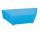Ethernet cez ulicu

Karol a Jozef sú vášniví hráči počítačových hier a býva v domoch, ktoré sú presne naproti sebe cez ulicu, takže si vidia navzájom do okien. Rozhodli sa, že si svoje počítače prepoja telefónnym káblom aby mohli hrať spoločne hry. Karol býva v prvom poschodí a jeho okno je vo výške 4,5 metra nad chodníkom. Jozef býva v treťom poschodí a okno má vo výške 10,1 metra.
Ulica je od domu k domu široká 10 metrov. Koľko metrov telefónneho kábla budú potrebovať pre toto spojenie?

Každý z nich si počíta, že bude mať počítač umiestnený v izbe asi 2 metre od okna.

Result

x =  15.5 m

Solution:Leave us a comment of example and its solution (i.e. if it is still somewhat unclear...):Be the first to comment!To solve this example are needed these knowledge from mathematics:

Do you want to convert length units? Pythagorean theorem is the base for the right triangle calculator. See also our trigonometric triangle calculator.

Next similar examples:

1. CablewayCableway has a length of 1800 m. The horizontal distance between the upper and lower cable car station is 1600 m. Calculate how much meters altitude is higher upper station than the base station.The ladder has a length 3.5 meters. He is leaning against the wall so that his bottom end is 2 meters away from the wall. Determine the height of the ladder.
3. Broken treeThe tree is broken at 4 meters above the ground and the top of the tree touches the ground at a distance of 5 from the trunk. Calculate the original height of the tree.
4. Center traverseIt is true that the middle traverse bisects the triangle?
5. Four ropesTV transmitter is anchored at a height of 44 meters by four ropes. Each rope is attached at a distance of 55 meters from the heel of the TV transmitter. Calculate how many meters of rope were used in the construction of the transmitter. At each attachment.8.3 meters long ladder is leaning against the wall of the well, and its lower end is 1.2 meters from this wall. How high from the bottom of a well is the top edge of the ladder?
7. StairwayStairway has 20 steps. Each step has a length of 22 cm and a height of 15 cm. Calculate the length of the handrail of staircases if on the top and bottom exceeds 10 cm.
8. BaseCompute base of an isosceles triangle, with the arm a=20 cm and a height above the base h=10 cm.Ladder 6.4 meters long is positioned in the well such that its lower end is distanced from the wall of the well 1.2 m. The upper part of the ladder is supported on the upper edge of the well. How high is the well?
10. Drainage channelThe cross section of the drainage channel is an isosceles trapezoid whose bases have a length of 1.80 m, 0.90 m and arm has length 0.60 meters. Calculate the depth of the channel.
11. Plane IIA plane flew 50 km on a bearing 63degrees20 and the flew on a bearing 153degrees20 for 140km. Find the distance between the starting point and the ending point
12. TriangleCan be rectangular triangle equilateral?
13. Spruce heightHow tall was spruce that was cut at an altitude of 8m above the ground and the top landed at a distance of 15m from the heel of the tree?
14. Broken treeThe tree was 35 meters high. The tree broke at a height of 10 m above the ground. Top but does not fall off it refuted on the ground. How far from the base of the tree lay its peak?
15. WindbreakA tree at a height of 3 meters broke in the windbreak. Its peak fell 4.5 m from the tree. How tall was the tree?
16. ThomasThomas lives 400 meters away from Samko, Robo from Thomas also 400 m and Samko from Robo 500. Anton lives 300 meters away from Robo further as Samko. How far away lives Anton from Rob?The double ladder is 8.5m long. It is built so that its lower ends are 3.5 meters apart. How high does the upper end of the ladder reach?